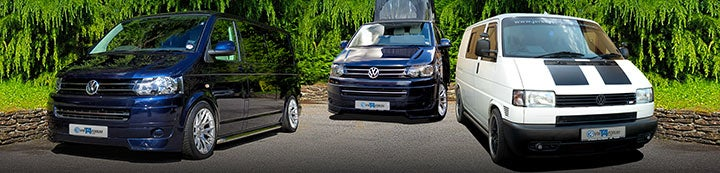1 - 4 of 4 Posts

#### StephenTazz

·
##### Registered
Joined
·
1,257 Posts
Discussion Starter
T4 Axle Weights

Choosing suitable tyres for the T4 that comply with United Kingdom and increasingly standardized Worldwide "Construction and Use" regulations can be problematic, especially when choosing tyres for non standard and oversized wheels.

The following reference guide summarizes the maximum permitted axle weight for each vehicle "group" in the T4 range...

T4a pre facelift - from August 1990 (M) to December 1995 (T) model years

Van and Window Van - nee Kombi (including Kombi based Campmobiles / lwb Eurovan Full Camper)

front axle weight
• 800 van (swb) = 1200kg
• 1000 (swb) = 1350kg
• 1200 (lwb) = 1430kg
rear axle weight
• 800 van (swb) = 1200kg
• 1000 (swb) = 1380kg
• 1200 (lwb) = 1460kg
Caravelle (including Eurovan, and Caravelle based swb Campmobiles)

• front axle weight = 1350kg
• rear axle weight = 1380kg
Chassis Cab (Eurovan Rialto and other "coachbuilt" models will have their own rear axle ratings)

• front axle weight = 1430kg
• rear axle weight = 1460kg
T4b post facelift - from January 1996 (T) to 2003 (3) model years

Van (including 1200 lwb Eurovan Full Camper)

front axle weight
• 800 van (swb only) = 1250kg
• 1000 (swb and lwb) = 1430kg
• 1200 (swb and lwb) = 1510kg
rear axle weight
• 800 van (swb only) = 1230kg
• 1000 (swb+ lwb) = 1410kg
• 1200 (swb+ lwb) = 1490kg
Caravelle (including Eurovan, Kombi, and Campmobiles)

front axle weight
• Caravelle/Eurovan (swb) = 1480kg
• Multivan, Kombi (swb) = 1510kg
• Caravelle, Kombi (lwb) = 1600kg
rear axle weight
• Multivan, Caravelle/Eurovan (swb) = 1230kg
• Kombi (swb) = 1330kg
• Caravelle (lwb) = 1330kg
• Kombi (lwb) = 1490kg
Chassis Cab (the Eurovan Railta and other "coachbuilt" models including the Razorback will have their own rear axle ratings)

• front axle weight = 1510kg
• rear axle weight = 1490kg
Footnote

Please note that VW guidelines to specialist coachbuilders allow for a +/- of 40kg on either corner; i.e an axle weight of 1500kg can be 750kg/750kg or 710kg/790kg so long as the overall weight remains at 1500kg.

#### Piplodocus

·
##### Registered
Joined
·
106 Posts
Just to clarify, does this mean if I have a "800 van (swb only) = 1250kg" this is the maximum weight per axle fully loaded?

So does this mean each wheel must take a weight of 1250kg divided by 2 = 625kg?

Hence each tyre and wheel must be rated for >625kg, and as long as it is I can load it up without any worries? :*

#### Dangermouse222

·
##### Registered
Joined
·
3 Posts
Just to clarify, does this mean if I have a "800 van (swb only) = 1250kg" this is the maximum weight per axle fully loaded?

So does this mean each wheel must take a weight of 1250kg divided by 2 = 625kg?

Hence each tyre and wheel must be rated for >625kg, and as long as it is I can load it up without any worries? :*
Is this correct?!:*

·